← Back to Index

# Quantization and sampling¶

Most signals in life are continuous: pressure waves propogating through air, chemical reactions, body movement. For computers to process these continuous signals, however, they must be converted to digital representations via a Analog-to-Digital Converter (ADC). A digital signal is different from its continous counterpart in two primary ways:

• It is sampled at specific time steps. For example, sound is often sampled at 44.1 kHz (or once every 0.023 milliseconds).
• It is quantized at specific voltage levels. For example, on the Arduino Uno, the microcontroller has a 10-bit ADC, so an incoming, continuous voltage input can be discretized at $\frac{5V}{2^{10}}=4.88 mV$ steps.

In this lesson, we will use audio data is our primary signal. Sound is a wonderful medium for learning because we can both visualize and hear the signal. Recall that a microphone responds to air pressure waves. We'll plot these waveforms, manipulate them, and then play them. We suggest plugging in your headphones, so you can really hear the distinctions in the various audio samples.

Note: We downsample audio data to 3,000 Hz and below. We could not get Chrome to play audio at these lower sampling rates (but they did work in Firefox). We'll make a note of this again when it's relevant.

## Dependencies¶

This notebook requires LibROSA—a python package for music and audio analysis. To install this package, you have two options.

First, from within Notebook, you can execute the following two lines within a cell (you'll only need to run this once):

import sys
!{sys.executable} -m pip install librosa

Second, from within your Anaconda shell:

> conda install -c conda-forge librosa

## Resources¶

This Notebook was designed and written by Professor Jon E. Froehlich at the University of Washington along with feedback from students. It is made available freely online as an open educational resource at the teaching website: https://makeabilitylab.github.io/physcomp/.

The website, Notebook code, and Arduino code are all open source using the MIT license.

Please file a GitHub Issue or Pull Request for changes/comments or email me directly.

# Main imports¶

In :
import librosa
import librosa.display
import IPython.display as ipd
import matplotlib.pyplot as plt # matplot lib is the premiere plotting lib for Python: https://matplotlib.org/
import numpy as np # numpy is the premiere signal handling library for Python: http://www.numpy.org/
import scipy as sp # for signal processing
from scipy import signal
import random
import makelab
from makelab import signal
from makelab import audio


# Quantization¶

Quantization refers to the process of transforming an analog signal, which has a continuous set of values, to a digital signal, which has a discrete set. See the figure below from Wikipedia's article on Quantization).

2-bit Quantization 3-bit Quantization2-bit resolution quantizes the analog signal into four levels ($2^{2}$) 3-bit resolution quantizes into eight levels ($2^{3}$)

The ATmega328 on the Arduino Uno, for example, has a 10-bit analog-to-digital (ADC) converter while the ESP32 has a 12-bit ADC. Because the ATmega328 runs on 5V, the ADC "step size" is $\frac{5V}{2^{10}} = 4.88mV$. This is the tiniest discriminable change you can observe on the Uno's analog input pins. In contrast, the ESP32 runs on 3.3V and has a higher bit resolution (12 bits), so the ADC has much finer discretizations: $\frac{3.3V}{2^{12}} = 0.806mV$—roughly, six times more precision than the Uno!

## Characterizing quantization error¶

A digitized sample can have a maximum error of one-half the discretization step size (i.e., ±½ the "Least Significant Bit" (LSB)). Why? Because when we convert an analog value to a digital one, we round to the nearest integer. Consider a voltage signal of 0.2271V on an Uno's analog input pin, this is nearly halfway between steps 0.2246V and 0.2295V, which would result in an error of $\frac{4.89mV}{2}$ (and either gets converted to 47 or 48 via Arduino's analogRead).

## Example: how does quantization affect audio signals?¶

For the examples below, we'll work with pre-digitalized audio waveforms sampled at 44.1kHz and quantized at 16-bits. So, while not a true continuous sample (of course not, it's already a digital signal!), we'll loosely treat it as such. And we'll "downsample" to investigate the effects of quantization levels and sampling rates.

Let's load, visualize, and play an initial 16-bit, 44.1kHz sound waveform of someone saying the word "Hello."

In :
# Feel free to change this wave file to any 16-bit audio sample

print(f"Sampling rate: {sampling_rate} Hz")
print(f"Number of channels = {len(audio_data_16bit.shape)}")
print(f"Total samples: {audio_data_16bit.shape}")

if len(audio_data_16bit.shape) == 2:
# convert to mono
audio_data_16bit = convert_to_mono(audio_data_16bit)

length_in_secs = audio_data_16bit.shape / sampling_rate
print(f"length = {length_in_secs}s")
print(audio_data_16bit)
quantization_bits = 16
print(f"{quantization_bits}-bit audio ranges from -{2**(quantization_bits - 1)} to {2**(quantization_bits - 1) - 1}")
print(f"Max value: {np.max(audio_data_16bit)} Avg value: {np.mean(audio_data_16bit):.2f}")

# We'll highlight and zoom in on the orange part of the graph controlled by xlim_zoom
xlim_zoom = (11000, 12500) # you may want to change this depending on what audio file you have loaded
makelab.signal.plot_signal(audio_data_16bit, sampling_rate, quantization_bits, xlim_zoom = xlim_zoom)
ipd.Audio(audio_data_16bit, rate=sampling_rate)

Sampling rate: 44100 Hz
Number of channels = 1
Total samples: 30833
length = 0.6991609977324263s
[-15 -15 -20 ... 158 144 153]
16-bit audio ranges from -32768 to 32767
Max value: 24125 Avg value: -3.82

Out: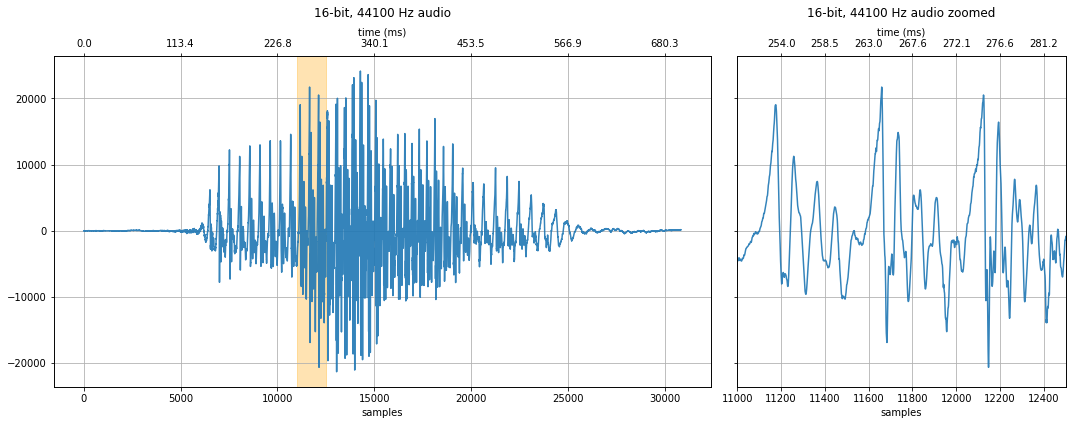### 8-bit quantization¶

We can convert the 16-bit audio down to other quantization levels to see and hear how quantization affects quality.

In :
# Convert to float
audio_data_float = audio_data_16bit / 2**16 # 16 bit audio

# With 8-bit audio, the voice still sounds pretty good
quantization_bits = 8
audio_data_8bit = audio_data_float * 2**quantization_bits
audio_data_8bit = audio_data_8bit.astype(int)
print(audio_data_8bit)
print(f"{quantization_bits}-bit audio ranges from -{2**(quantization_bits - 1)} to {2**(quantization_bits - 1) - 1}")
print(f"Max value: {np.max(audio_data_8bit)} Avg value: {np.mean(audio_data_8bit):.2f}")

makelab.signal.plot_signal(audio_data_8bit, sampling_rate, quantization_bits, xlim_zoom = xlim_zoom)
ipd.Audio(audio_data_8bit, rate=sampling_rate)

[0 0 0 ... 0 0 0]
8-bit audio ranges from -128 to 127
Max value: 94 Avg value: 0.06

Out: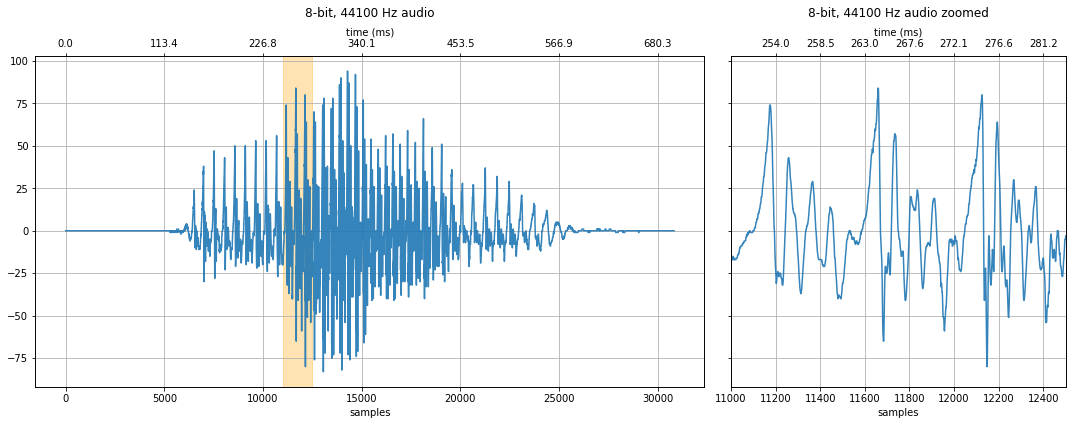With 8-bit quantization, the y-axis ranges from -128 to 127. Look closely at the waveform, can you notice any differences with 16-bit audio? How about when you listen to the 8-bit vs. 16-bit version?

### 6-bit quantization¶

How about 6-bits? At this level, we can start to hear degradations in the signal—a hissing sound (at least with headphones). And we can begin to see obvious discretized steps in the zoomed-in waveform.

In :
quantization_bits = 6
audio_data_6bit = audio_data_float * 2**quantization_bits
audio_data_6bit = audio_data_6bit.astype(int)
print(audio_data_6bit)
print(f"{quantization_bits}-bit audio ranges from -{2**(quantization_bits - 1)} to {2**(quantization_bits - 1) - 1}")
print(f"Max value: {np.max(audio_data_6bit)} Avg value: {np.mean(audio_data_6bit):.2f}")

makelab.signal.plot_signal(audio_data_6bit, sampling_rate, quantization_bits, xlim_zoom = xlim_zoom)
ipd.Audio(audio_data_6bit, rate=sampling_rate)

[0 0 0 ... 0 0 0]
6-bit audio ranges from -32 to 31
Max value: 23 Avg value: 0.06

Out: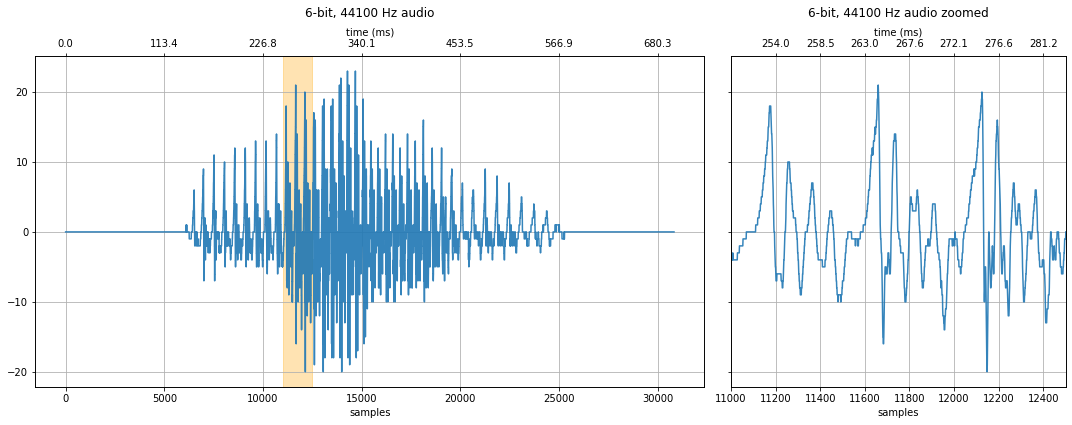### 4-bit quantization¶

At 4 bits, the noise is more substantial. Take a look at the zoom plot on the right, the "steps" between quantization levels are far more noticeable. And yet, our hears can still somehow parse the word "hello"—though you should playback this signal for someone who doesn't know what's being said to determine comprehensibility.

In :
quantization_bits = 4
audio_data_4bit = audio_data_float * 2**quantization_bits
audio_data_4bit = audio_data_4bit.astype(int)
print(audio_data_4bit)
print(f"{quantization_bits}-bit audio ranges from -{2**(quantization_bits - 1)} to {2**(quantization_bits - 1) - 1}")
print(f"Max value: {np.max(audio_data_4bit)} Avg value: {np.mean(audio_data_4bit):.2f}")

makelab.signal.plot_signal(audio_data_4bit, sampling_rate, quantization_bits, xlim_zoom = xlim_zoom)
ipd.Audio(audio_data_4bit, rate=sampling_rate)

[0 0 0 ... 0 0 0]
4-bit audio ranges from -8 to 7
Max value: 5 Avg value: 0.05

Out: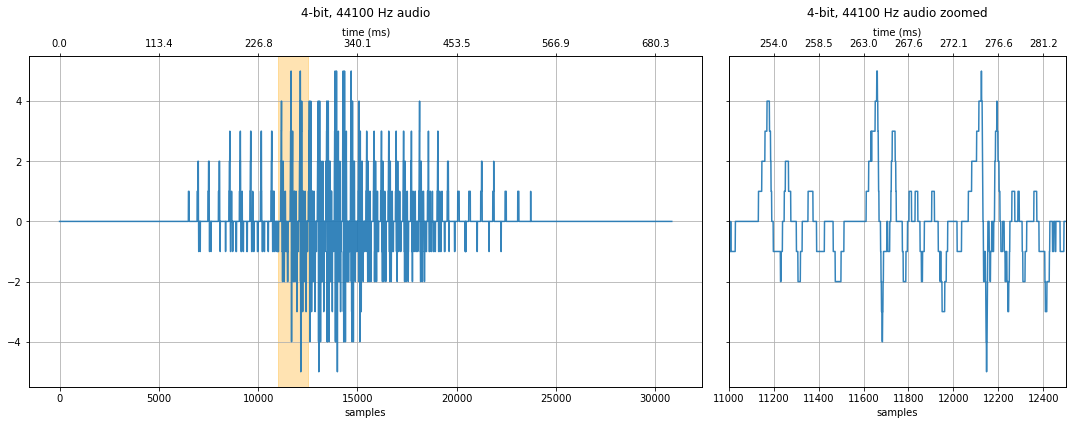### 3-bit quantization¶

At 3-bits, the sound is no longer intelligible—at least not if you didn't already know what the audio sample was saying. What parts of the degraded signal are still perceptible? There is still an observable "rhythm" to the sound.

In :
# 3-bit audio
quantization_bits = 3
audio_data_3bit = audio_data_float * 2**quantization_bits
audio_data_3bit = audio_data_3bit.astype(int)
print(audio_data_3bit)
print(f"{quantization_bits}-bit audio ranges from -{2**(quantization_bits - 1)} to {2**(quantization_bits - 1) - 1}")
print(f"Max value: {np.max(audio_data_3bit)} Avg value: {np.mean(audio_data_3bit):.2f}")

fig, axes = makelab.signal.plot_signal(audio_data_3bit, sampling_rate, quantization_bits, xlim_zoom = xlim_zoom)
major_ticks = np.arange(-4, 5, 1)
axes.set_yticks(major_ticks)
axes.set_yticks(major_ticks)
ipd.Audio(audio_data_3bit, rate=sampling_rate)

[0 0 0 ... 0 0 0]
3-bit audio ranges from -4 to 3
Max value: 2 Avg value: 0.03

Out: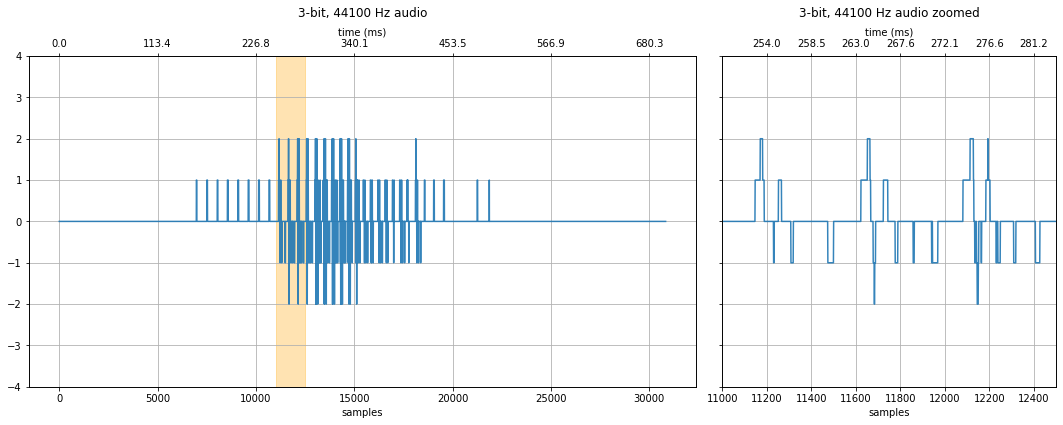### 2-bit quantization¶

What if we try 2-bit audio? That's only four quantization levels!

In :
# 2-bit audio
quantization_bits = 2
audio_data_2bit = audio_data_float * 2**quantization_bits
audio_data_2bit = audio_data_2bit.astype(int)
print(audio_data_2bit)
print(f"{quantization_bits}-bit audio ranges from -{2**(quantization_bits - 1)} to {2**(quantization_bits - 1) - 1}")
print(f"Max value: {np.max(audio_data_2bit)} Avg value: {np.mean(audio_data_2bit):.2f}")

fig, axes = makelab.signal.plot_signal(audio_data_2bit, sampling_rate, quantization_bits, xlim_zoom = xlim_zoom)
#axes.grid(ydata=[0, 1])
major_ticks = np.arange(-2, 3, 1)
axes.set_yticks(major_ticks)
axes.set_yticks(major_ticks)
ipd.Audio(audio_data_2bit, rate=sampling_rate)

[0 0 0 ... 0 0 0]
2-bit audio ranges from -2 to 1
Max value: 1 Avg value: 0.00

Out: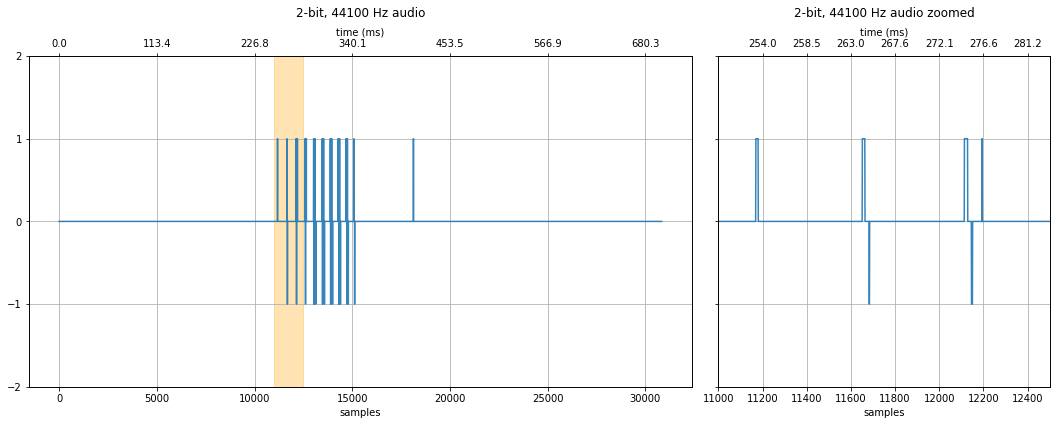## Exercise: play with your own audio data and quantization¶

In :
# Change this wave file to any 16-bit audio sample
your_sound_file = 'data/audio/Guitar_MoreThanWords_16bit_44.1kHz_stereo.wav'

print(f"Sampling rate: {your_sampling_rate} Hz")
print(f"Number of channels = {len(your_audio_data_16_bit.shape)}")
print(f"Total samples: {your_audio_data_16_bit.shape}")

if len(your_audio_data_16_bit.shape) == 2:
# convert to mono
print("Converting stereo audio file to mono")
your_audio_data_16_bit = your_audio_data_16_bit.sum(axis=1) / 2

# Convert to float
your_audio_data_float = your_audio_data_16_bit / 2**16 # 16 bit audio

# Try different quantization levels here
quantization_bits = 6 # change this and see what happens!
your_audio_data_quantized = your_audio_data_float * 2**quantization_bits
your_audio_data_quantized = your_audio_data_quantized.astype(int)
print(your_audio_data_quantized)
print(f"{quantization_bits}-bit audio ranges from -{2**(quantization_bits - 1)} to {2**(quantization_bits - 1) - 1}")
print(f"Max value: {np.max(your_audio_data_quantized)} Avg value: {np.mean(your_audio_data_quantized):.2f}")

xlim_zoom = (46000, 50000) # make sure to change the zoom range too
makelab.signal.plot_signal(your_audio_data_16_bit, sampling_rate, 16, xlim_zoom = xlim_zoom)
makelab.signal.plot_signal(your_audio_data_quantized, sampling_rate, quantization_bits, xlim_zoom = xlim_zoom)
ipd.Audio(your_audio_data_quantized, rate=sampling_rate)

Sampling rate: 44100 Hz
Number of channels = 2
Total samples: 1096151
Converting stereo audio file to mono
[0 0 0 ... 0 0 0]
6-bit audio ranges from -32 to 31
Max value: 26 Avg value: 0.01

Out: